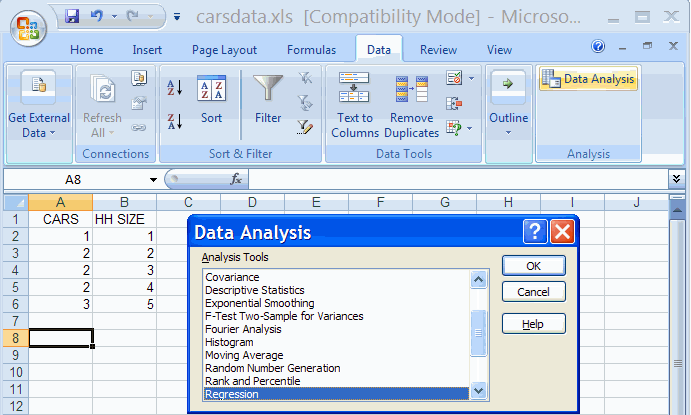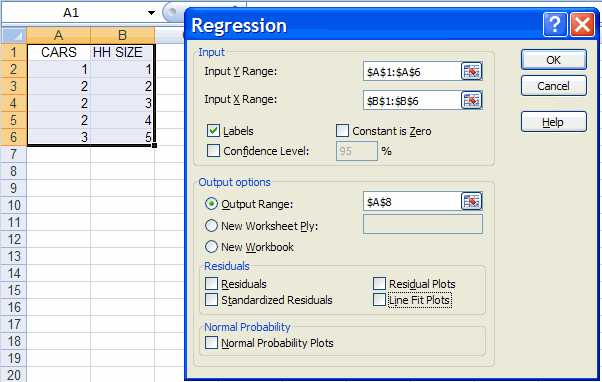## A. Colin Cameron, Dept. of Economics, Univ. of Calif. - Davis

This January 2009 help sheet gives information on
• Two-variable linear regression.
• Run the regression using the Data Analysis Add-in.
• Interpreting the regression summary output (but not performing statistical inference).
This handout is the first place to go to for two-variable regression output.
After reading this move onto Excel 2007: Statistical Inference for Two-Variable Regression

Other ways to do two-variable regression are discussed
in Excel 2007: Two-way Plots in the section on Add a trendline and
in Excel 2007: Two Variable Regression using Functions LINEST

TWO-VARIABLE LINEAR REGRESSION

The population regression model is:    y = β1 + β2 x + u

We wish to estimate the regression line:     y = b1 + b2 x

REGRESSION USING THE DATA ANALYSIS ADD-IN

This requires the Data Analysis Add-in: see Excel 2007: Access and Activating the Data Analysis Add-in

The data used are in carsdata.xls

• In the Data Group select the Data Analysis Add-in
• Select Regression AnalysisWe select OK and fill out the dialog box as followsWe obtainINTERPRETING THE REGRESSION SUMMARY OUTPUT

The key output is given in the Coefficients column in the last set of output:
• b1 = 0.8     (the Intercept coefficient)
• b2 = 0.4     (the Coefficient of HH SIZE : the slope coefficient)
Thus the fitted line is:     y = 0.8 + 0.4 x
or                             CARS = 0.8 + 0.4 HHSIZE

The regression statistics outyput gives measures of how well the model fits the data. In particular

• R2 = 0.8   which measures the fit of the model
This means that 80% of the variation of yi around ybar is explained by the regressor xi
• Standard error = 0.365 which measures the standard deviation of yi around its fitted value.

The remaining output (ANOVA table and t Stat, p-value, .... ) is used for statistical inference.
See  Excel 2007: Statistical Inference for Two-Variable Regression

For further information on how to use Excel go to
http://cameron.econ.ucdavis.edu/excel/excel.html